#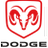Dodge Durango Service ManualTransmission and Transfer Case » Description and operationPistons

DESCRIPTION

There are several sizes and types of pistons used in an automatic transmission. Some pistons are used to apply clutches, while others are used to apply bands.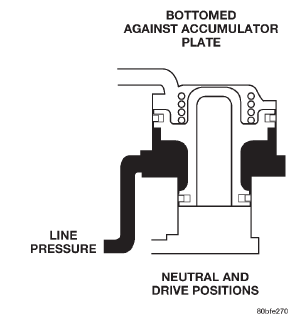Fig. 48 Accumulator in Neutral and Drive Positions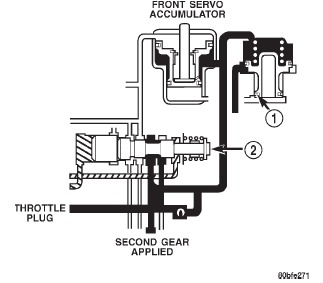Fig. 49 Accumulator in Second Gear Position

1 - BOTTOM IN BORE

2 - SHUTTLE VALVE

They all have in common the fact that they are round or circular in shape, located within a smooth walled cylinder, which is closed at one end and converts fluid pressure into mechanical movement. The fluid pressure exerted on the piston is contained within the system through the use of piston rings or seals.

OPERATION

The principal which makes this operation possible is known as Pascal's Law. Pascal's Law can be stated as: "Pressure on a confined fluid is transmitted equally in all directions and acts with equal force on equal areas."

PRESSURE

Pressure (Fig. 50) is nothing more than force (lbs.) divided by area (in or ft.), or force per unit area.

Given a 100 lb. block and an area of 100 sq. in. on the floor, the pressure exerted by the block is: 100 lbs. 100 in or 1 pound per square inch, or PSI as it is commonly referred to.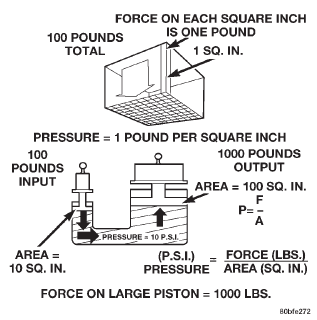Fig. 50 Force and Pressure Relationship

PRESSURE ON A CONFINED FLUID

Pressure is exerted on a confined fluid (Fig. 51) by applying a force to some given area in contact with the fluid. A good example of this is a cylinder filled with fluid and equipped with a piston that is closely fitted to the cylinder wall. If a force is applied to the piston, pressure will be developed in the fluid. Of course, no pressure will be created if the fluid is not confined. It will simply "leak" past the piston. There must be a resistance to flow in order to create pressure.

Piston sealing is extremely important in hydraulic operation. Several kinds of seals are used to accomplish this within a transmission. These include but are not limited to O-rings, D-rings, lip seals, sealing rings, or extremely close tolerances between the piston and the cylinder wall. The force exerted is downward (gravity), however, the principle remains the same no matter which direction is taken.

The pressure created in the fluid is equal to the force applied, divided by the piston area. If the force is 100 lbs., and the piston area is 10 sq. in., then the pressure created equals 10 PSI. Another interpretation of Pascal's Law is that regardless of container shape or size, the pressure will be maintained throughout, as long as the fluid is confined. In other words, the pressure in the fluid is the same everywhere within the container.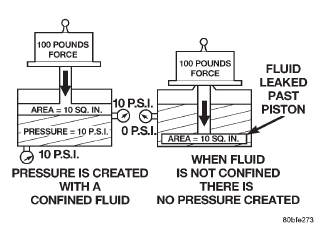Fig. 51 Pressure on a Confined Fluid

FORCE MULTIPLICATION

Using the 10 PSI example used in the illustration (Fig. 52), a force of 1000 lbs. can be moved with a force of only 100 lbs. The secret of force multiplication in hydraulic systems is the total fluid contact area employed. The illustration, (Fig. 52), shows an area that is ten times larger than the original area.

The pressure created with the smaller 100 lb. input is 10 PSI. The concept "pressure is the same everywhere" means that the pressure underneath the larger piston is also 10 PSI. Pressure is equal to the force applied divided by the contact area. Therefore, by means of simple algebra, the output force may be found. This concept is extremely important, as it is also used in the design and operation of all shift valves and limiting valves in the valve body, as well as the pistons, of the transmission, which activate the clutches and bands. It is nothing more than using a difference of area to create a difference in pressure to move an object.

PISTON TRAVEL

The relationship between hydraulic lever and a mechanical lever is the same. With a mechanical lever it's a weight-to-distance output rather than a pressure-to-area output. Using the same forces and areas as in the previous example, the smaller piston (Fig. 53) has to move ten times the distance required to move the larger piston one inch. Therefore, for every inch the larger piston moves, the smaller piston moves ten inches. This principle is true in other instances also. A common garage floor jack is a good example. To raise a car weighing 2000 lbs., an effort of only 100 lbs. may be required. For every inch the car moves upward, the input piston at the jack handle must move 20 inches downward.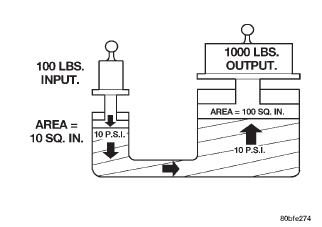Fig. 52 Force Multiplication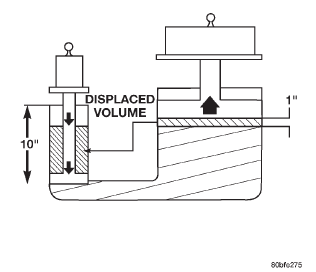Fig. 53 Piston Travel

## Categories